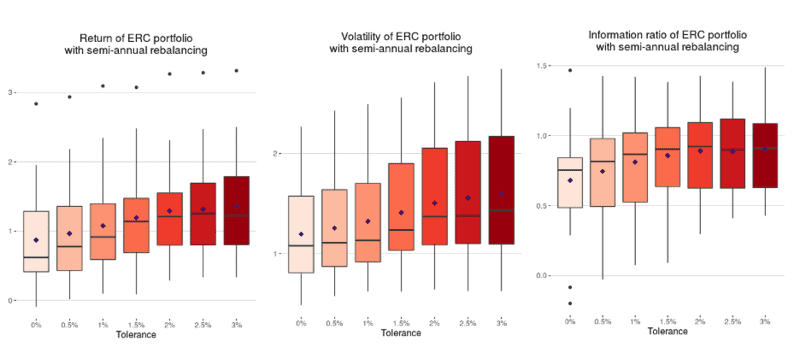# Tolerance Thresholds: Avoiding Costly Portfolio Rebalancing

Including a tolerance threshold – which means a security or strategy does not need to be reweighted in a portfolio in some cases – can improve information ratios with only a marginal increase in volatility.

A report by Quantilia quantitative analyst suggests the most useful tolerance threshold range is between 2% and 3%, based on a study of equal-risk-contribution (ERC), inverse-volatility and equal-weighting portfolio schemes.

Tolerance thresholds work by allowing the weight of a security, fund or strategy in a portfolio to drift slightly away from its targeted weight without having to reweight it. This reduces portfolio turnover and saves on trading costs. If the strategy’s weight drifts too far, and breaches the tolerance threshold, then it will need to be reweighted at the next portfolio rebalancing interval.

For example, if the current weight of a strategy in a portfolio is 6%, the targeted weight is 7% and the tolerance threshold is 2%, then the strategy will not be reweighted since its weighting has only drifted by one percentage point.

The Quantilia study finds that including a tolerance threshold can improve the information ratios of ERC, inverse-volatility and equally-weighted portfolios, with only a small increase in volatility.The information ratio measures excess returns per unit of volatility risk, essentially gauging how consistent a strategy’s outperformance over a benchmark is. The higher the information ratio, the better.

Quantilia’s report was based on 20 baskets of 20 strategies each, with a split between alpha and beta strategies and exposure to different asset classes and strategy providers. The study used a semi-annual rebalancing approach and assumed transaction costs to be 20 basis points.

ERC funds are weighted in such a way that each security contributes equally to total portfolio risk, thereby reducing the risk concentration of the portfolio. This is similar to the inverse-volatility approach, though the ERC strategy also accounts for the correlations between securities within the portfolio.

With an increase of tolerance thresholds, the value of average portfolio turnover decreased under all weighting schemes studied. Several equally-weighted portfolios even had zero portfolio turnover with a threshold of 3%.